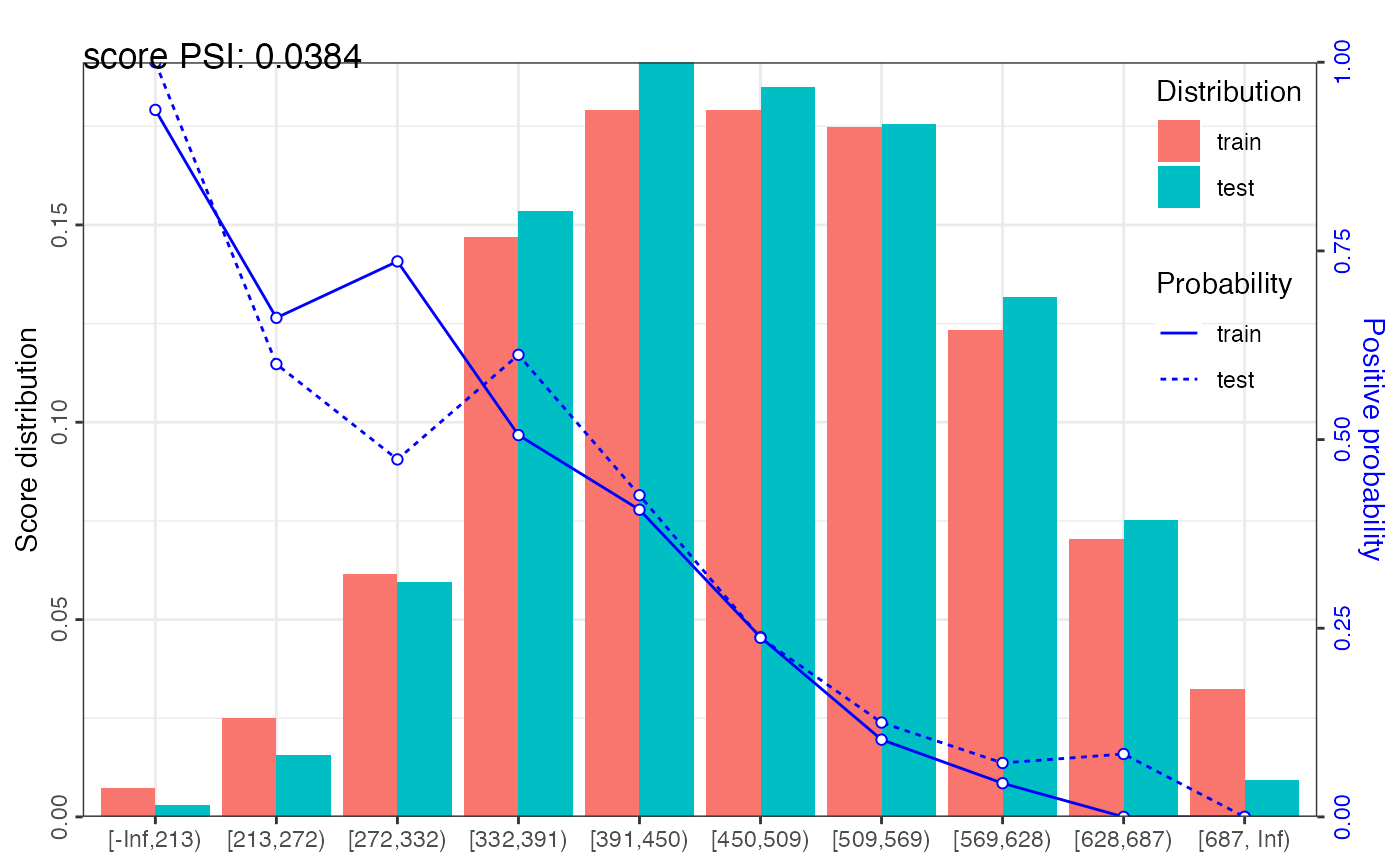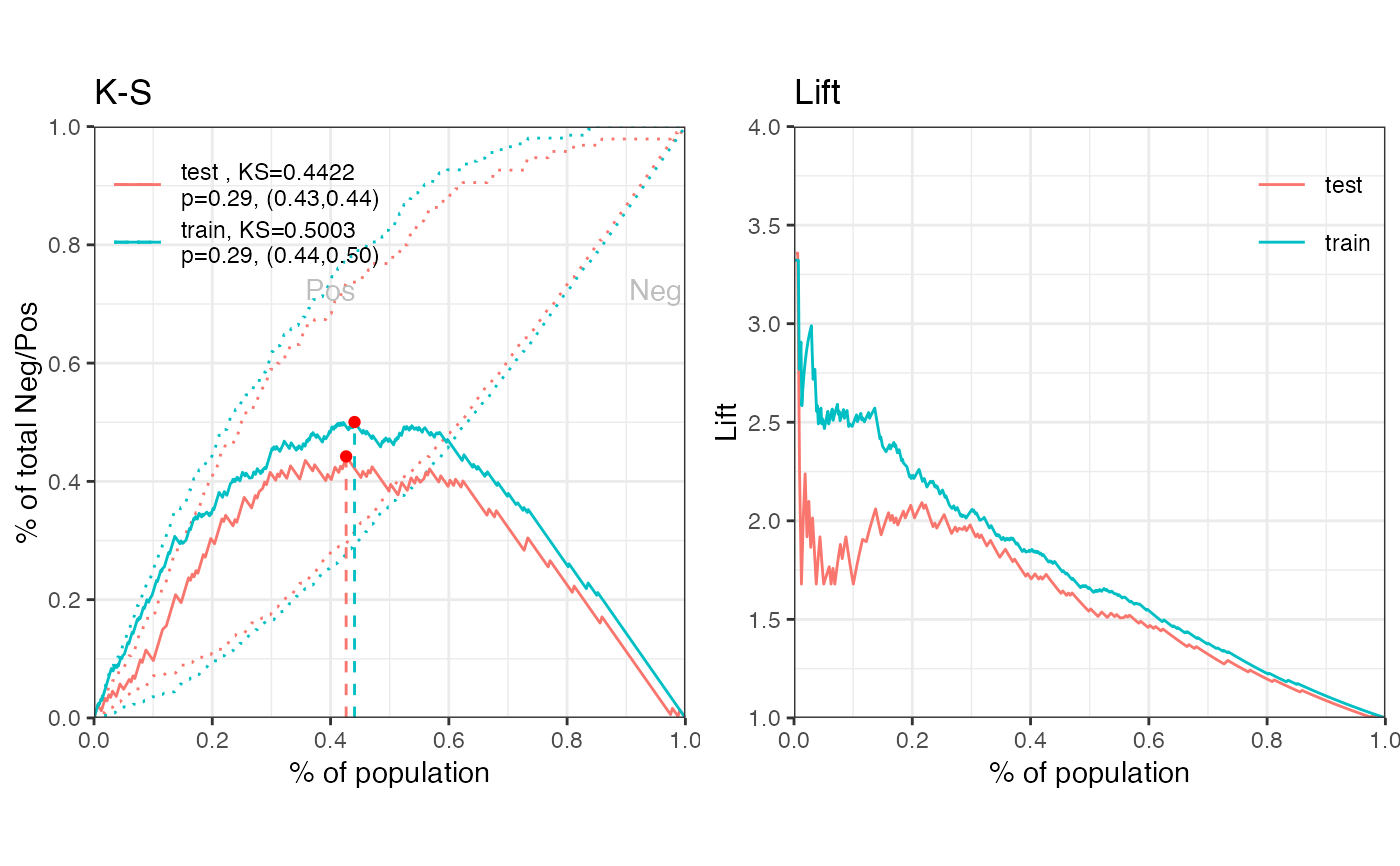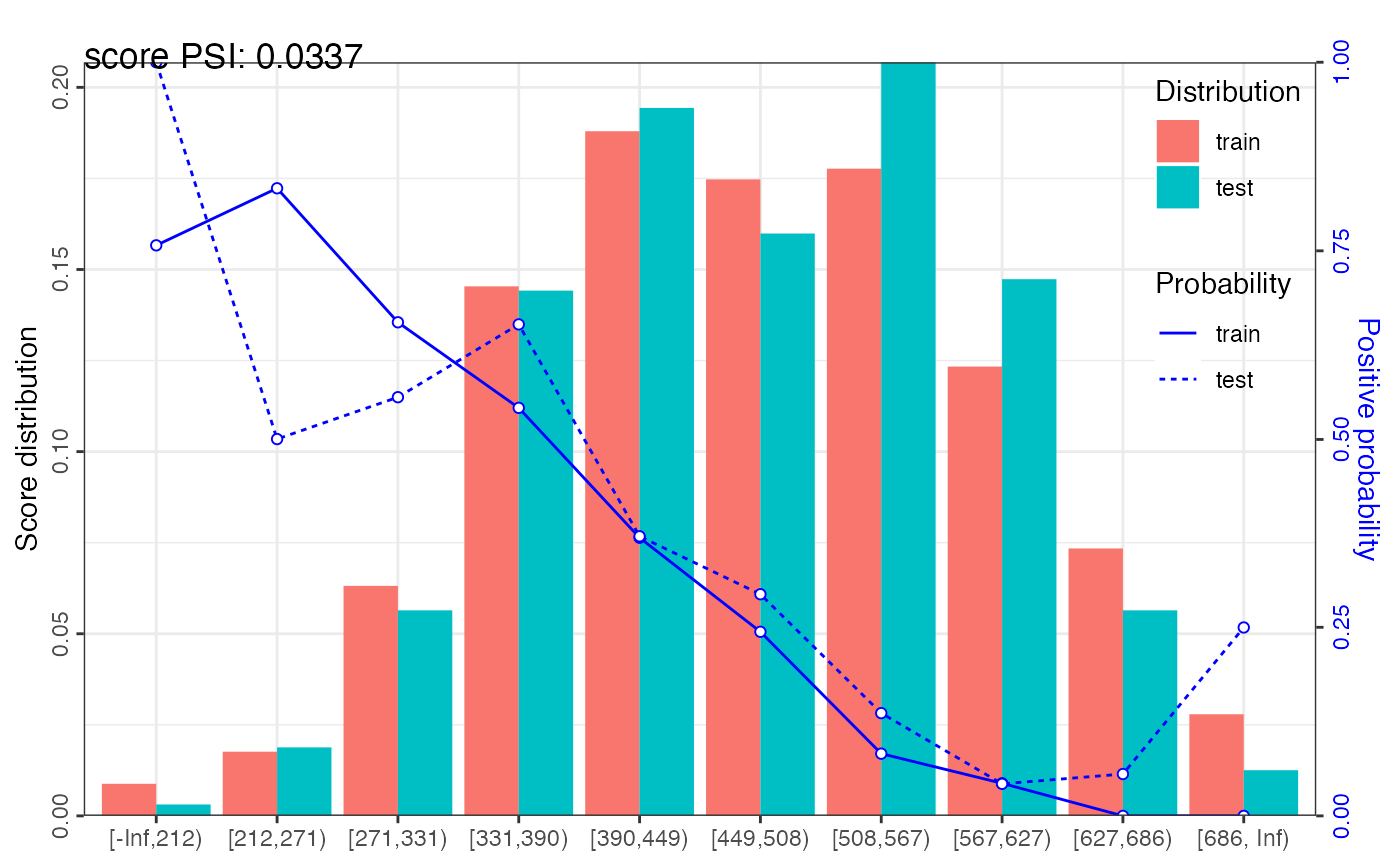perf_psi calculates population stability index (PSI) for total credit score and Characteristic Stability Index (CSI) for variables. It can also creates graphics to display score distribution and positive rate trends.

perf_psi(score, label = NULL, title = NULL, show_plot = TRUE,
positive = "bad|1", threshold_variable = 20, var_skip = NULL, ...)

## Arguments

score A list of credit score for actual and expected data samples. For example, score = list(expect = scoreE, actual = scoreA). A list of label value for actual and expected data samples. For example, label = list(expect = labelE, actual = labelA). Defaults to NULL. Title of plot, Defaults to NULL. Logical. Defaults to TRUE. Value of positive class, Defaults to "bad|1". Integer. Defaults to 20. If the number of unique values > threshold_variable, the provided score will be counted as total credit score, otherwise, it is variable score. Name of variables that are not score, such as id column. It should be the same with the var_kp in scorecard_ply function. Defaults to NULL. Additional parameters.

## Value

A data frame of psi and graphics of credit score distribution

## Details

The population stability index (PSI) formula is displayed below: $$PSI = \sum((Actual\% - Expected\%)*(\ln(\frac{Actual\%}{Expected\%}))).$$ The rule of thumb for the PSI is as follows: Less than 0.1 inference insignificant change, no action required; 0.1 - 0.25 inference some minor change, check other scorecard monitoring metrics; Greater than 0.25 inference major shift in population, need to delve deeper.

Characteristic Stability Index (CSI) formula is displayed below: $$CSI = \sum((Actual\% - Expected\%)*score).$$

perf_eva gains_table

## Examples

# \donttest{
# data preparing ------
data("germancredit")
# filter variable via missing rate, iv, identical value rate
dt_f = var_filter(germancredit, "creditability")
#> [INFO] filtering variables ... # breaking dt into train and test
dt_list = split_df(dt_f, "creditability")
label_list = lapply(dt_list, function(x) x$creditability) # woe binning ------ bins = woebin(dt_list$train, "creditability")
#> [INFO] creating woe binning ... # converting train and test into woe values
dt_woe_list = lapply(dt_list, function(x) woebin_ply(x, bins))
#> [INFO] converting into woe values ...
#> [INFO] converting into woe values ...
# glm ------
m1 = glm(creditability ~ ., family = binomial(), data = dt_woe_list$train) # vif(m1, merge_coef = TRUE) # Select a formula-based model by AIC m_step = step(m1, direction="both", trace=FALSE) m2 = eval(m_step$call)
# vif(m2, merge_coef = TRUE)

# predicted proability
pred_list = lapply(dt_woe_list, function(x) predict(m2, type = 'response', x))

# scorecard ------
card = scorecard(bins, m2)

# credit score, only_total_score = TRUE
score_list = lapply(dt_list, function(x) scorecard_ply(x, card))
# credit score, only_total_score = FALSE
score_list2 = lapply(dt_list, function(x) scorecard_ply(x, card, only_total_score=FALSE))

###### perf_eva examples ######
# Example I, one datset
## predicted p1
perf_eva(pred = pred_list$train, label=dt_list$train$creditability, title = 'train')#>$binomial_metric
#> $binomial_metric$train
#>          MSE      RMSE   LogLoss        R2        KS       AUC      Gini
#> 1: 0.1519266 0.3897776 0.4578621 0.2779502 0.5003279 0.8267473 0.6534946
#>
#>
#> $pic #> TableGrob (1 x 2) "arrange": 2 grobs #> z cells name grob #> 1 1 (1-1,1-1) arrange gtable[layout] #> 2 2 (1-1,2-2) arrange gtable[layout] #> ## predicted score # perf_eva(pred = score_list$train, label=dt_list$train$creditability, title = 'train')

# Example II, multiple datsets
## predicted p1
perf_eva(pred = pred_list, label = label_list)#> $binomial_metric #>$binomial_metric$train #> MSE RMSE LogLoss R2 KS AUC Gini #> 1: 0.1519266 0.3897776 0.4578621 0.2779502 0.5003279 0.8267473 0.6534946 #> #>$binomial_metric$test #> MSE RMSE LogLoss R2 KS AUC Gini #> 1: 0.1731937 0.4161655 0.522526 0.1717874 0.4421992 0.7714991 0.5429981 #> #> #>$pic
#> TableGrob (1 x 2) "arrange": 2 grobs
#>   z     cells    name           grob
#> 1 1 (1-1,1-1) arrange gtable[layout]
#> 2 2 (1-1,2-2) arrange gtable[layout]
#> ## predicted score
# perf_eva(score_list, label_list)

###### perf_psi examples ######
# Example I # only total psi
psi1 = perf_psi(score = score_list, label = label_list)
psi1$psi # psi data frame #> variable dataset psi #> 1: score train_test 0.03371411psi1$pic  # pic of score distribution
#> $score#> # modify colors # perf_psi(score = score_list, label = label_list, # line_color='#FC8D59', bar_color=c('#FFFFBF', '#99D594')) # Example II # both total and variable psi psi2 = perf_psi(score = score_list2, label = label_list) # psi2$psi  # psi data frame
# psi2$pic # pic of score distribution ###### gains_table examples ###### # Example I, input score and label can be a list or a vector g1 = gains_table(score = score_list$train, label = label_list\$train)
g2 = gains_table(score = score_list, label = label_list)

# Example II, specify the bins number and type
g3 = gains_table(score = score_list, label = label_list, bin_num = 20)
g4 = gains_table(score = score_list, label = label_list, method = 'width')
# }